#### Generating a random element of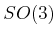One has to be very careful about sampling uniformly over the space of rotations. The probability density must correspond to the Haar measure, which means that a random rotation should be obtained by picking a point at random on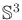and forming the unit quaternion. An extremely clever way to sampleuniformly at random is given in  and is reproduced here. Choose three points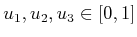uniformly at random. A uniform, random quaternion is given by the simple expression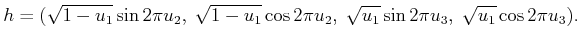(5.15)

A full explanation of the method is given in , and a brief intuition is given here. First drop down a dimension and pick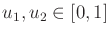to generate points on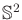. Let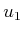represent the value for the third coordinate,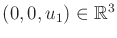. The slice of points onfor whichis fixed for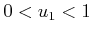yields a circle onthat corresponds to some line of latitude on. The second parameter selects the longitude,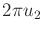. Fortunately, the points are uniformly distributed over. Why? Imagineas the crust on a spherical loaf of bread that is run through a bread slicer. The slices are cut in a direction parallel to the equator and are of equal thickness. The crusts of each slice have equal area; therefore, the points are uniformly distributed. The method proceeds by using that fact thatcan be partitioned into a spherical arrangement of circles (known as the Hopf fibration); there is an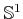copy for each point in. The method above is used to provide a random point onusing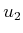and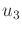, andproduces a random point on; they are carefully combined in (5.15) to yield a random rotation. To respect the antipodal identification for rotations, any quaternion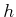found in the lower hemisphere (i.e.,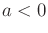) can be negated to yield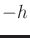. This does not distort the uniform random distribution of the samples.

Steven M LaValle 2020-08-14